# Crack the top 40 Java coding interview questions

Nov 05, 2020 - 25 min readRyan ThelinJava remains a popular language across the world. Especially in financial fields, Java’s scalability and efficiency keep it in-demand with interviewers in a variety of famous companies like Goldman Sachs, eBay, and Google.

Today, we’ll help you prepare for your upcoming coding interview at these and other popular eCommerce companies by reviewing the top 40 Java questions asked by interviewers. By the end, you’ll have the experience to walk into any Java interview with confidence.

Let’s get started!

Here are the types of questions we’ll cover today:

## 1. Nested Loop with Addition

Find the Big O complexity of the following code:

class NestedLoop {
public static void main(String[] args) {
int n = 10; // 1 step --> Note: n can be anything. This is just an example
int sum = 0; // 1 step
double pie = 3.14; // 1 step

for (int var = 0; var < n; var = var + 3) { // n/3 steps
System.out.println("Pie: " + pie); // n/3 steps
for (int j = 0; j < n; j = j + 2) {  // (n/3 * n/2) steps
sum++;   // (n/3 * n/2) steps
System.out.println("Sum = " + sum);  // (n/3 * n/2) steps
}
}
}
}


Solution Explanation:

The first for loop on line 7 can be broken down into 3 parts:

• initialization
• comparison
• incrementation

Since the initialization (int var = 0) only happens once in the entire program, it takes 1 unit of time. The comparison (var < n) gets executed $\frac{n}{3} + 1$ times and the increment runs $\frac{n}{3}$ times.

Similarly, (int j = 0) runs $\frac{n}{3} times , the comparison (j < n) runs $n/3 \times$ $\frac{n}{2}$ $+ 1$. The test case runs once more than the whole loop where boundary check fails! The increment (j = j + 2) gets executed $\frac{n}{2}$ times for each iteration of the outer loop–which makes it run a total of $\frac{n}{3} \times \frac{n}{2} = \frac{n^2}{6}$ times. Run time complexity: $\frac{(15+5n+2n^2)} {3}$ To find Big O, we: • Drop leading constants • Drop lower order terms Therefore, Big O Complexity: $O(n^2)$ ## 2. Nested Loop with Multiplication Find the Big O complexity of the following code segment: class NestedLoop { public static void main(String[] args) { int n = 10; // O(time complexity of the called function) int sum = 0; //O(1) double pie = 3.14; //O(1) int var = 1; while(var < n) { // O(log n) System.out.println("Pie: " + pie); // O(log n) for (int j = 0; j < var; j++) { // 2n sum++; // (2n-1) } var *= 2; // O(log n) } //end of while loop System.out.println("Sum: " + sum); //O(1) } //end of main } //end of class  Solution Explanation: A loop statement that multiplies/divides the loop variable by a constant takes$\log_k(n) time because the loop runs that many times. In the outer loop, the loop variable is multiplied by 2 in each iteration. Therefore, the outer loop runs $O(log_2 \space n)$ times.

The inner loop runs var times, not n times. The value of var is 1 in the first iteration, then 2, then 4, then 8, and so on until 2^ksuch that 2^k < 2. So when we sum the var values for all the iterations of the outer loop, the inner loop runs $2^k$ times. We’ll use a geometric series to figure out this value. To make this calculation simpler, let’s assume that $2^k = n$:

$2^{k+1} - 1$

$2^k2^1 - 1$

Substituting n for $2^k$ we get:

= $2n-1$

So it appears that the inner loop runs a total of $2n-1$ times (Considering all the iterations of the outer loop), but remember that we assumed that $n = 2^k$ when $n>2^k$ so, in actuality, the inner loop runs less than $2n-1$ times, but we can consider this to be the upper bound.

Runtime Complexity: $4+4log_ 2(n)+6n$

Big O: $O(n)$

## 3. Nested Loop with Multiplication (Advanced)

Find the Big O Complexity of the following code segment:

 class NestedLoop {
public static void main(String[] args) {
int n = 10;
int sum = 0;
double pie = 3.14;

for (int var = 0; var < n; var++) {
int j = 1;
System.out.println("Pie: " + pie);
while(j < var) {
sum += 1;
j *= 2;
}
} //end of for loop
System.out.println("Sum: " + sum);
} //end of main
} //end of class


Solution Explanation:

In the main function, the outer loop is $O(n)$ as it iterates n times. The inner while loop iterates var times, which is always less than n and the inner loop counter variable is doubled each time. Therefore we can say that it is $O(log_2(n))$. Thus, the total time complexity of the program given above becomes:

$O(nlog_2(n))$

The running time complexity mentioned above is a loose bound. To evaluate a tighter bound on the running time of the program given above, let’s look at the inner loop once again.

The inner loop depends upon j which is less than var and is multiplied by 2 in each iteration. This means that the complexity of the inner loop is $O(log_2(\text{var}))$. But, the value of var at each iteration, of the outer loop, is different. The total complexity of the inner loop in terms of n can be calculated as such:

$n \times log_2(var)$

$\Rightarrow\sum_{var=1}^{n} log_2 (var)$

$\Rightarrow log_2 (1) +... + log_2 (n-1) + log_2 (n)$

Further, we know:

$log(a) + log(b) = log(ab)$

Therefore, the above expression becomes:

$\Rightarrow log_2 (1 \times 2 \times ... \times (n-1) \times(n) )$

$\Rightarrow log_2 (n!)$

Thus, the total time complexity of the inner-loop (considering the outer-loop) is $O(log_2(n!))$.

Time complexity: $7+4n+3log_​2 (n!)$

Big O: $O(log_2(n!))$.

This is a tight upper-bound to the growth function of this program. And as mentioned above, the loose upper bound is $O(nlog_2(n))$.

## 4. Conditional Loop

What is the Big O complexity of the following code segment:

    void complexMethod(int[] array) {
int n = array.length;
int runFor = Math.pow(-1, n) * Math.pow(n, 2);
for (int i = 0; i < runFor; i++) {
System.out.println("Find how complex I am ?")
}
}


Solution Explanation:

A common mistake with this question is to say that the complexity is $O(n)$. This is incorrect because the worst case only happens for even sizes of the input array.

The loop doesn’t run when the size of the array is an odd number.

Next, the loop runs a quadratic number of times the length of the array when the length is even.

The bigger the array size and provided it is an even number, the single loop runs as if it were a nesting of two loops.

Therefore, the complexity of this snippet of code is $O(n^2)$.

## 5. Heavily nested loops

Find the Big O complexity of the following code segment:

    void someMethod(int n, int m) {

for (int j = 0; j < n; j++) {
for (int i = 0; i < 3; i++) {
for (int i = 0; i < n; i++) {
System.out.println("I have 3 loops");
}
}
}
}


Solution Breakdown:

The best way to solve this problem is to unroll the second loop. Here’s what this code would look like unrolled:

    void someMethod(int n) {

for (int j = 0; j < n; j++) {
for (int i = 0; i < n; i++) {
System.out.println("I have 3 loops");
}
for (int i = 0; i < n; i++) {
System.out.println("I have 3 loops");
}
for (int i = 0; i < n; i++) {
System.out.println("I have 3 loops");
}
}
}


From the unrolling, it is evident that the three inner loops contribute $n + n + n = 3n = O(n)$ and the outermost loop runs for n too, therefore the overall complexity is $O(n^2)$.

## Data Structures

### 6. Array of Products of All Elements Except Itself

Problem Statement:

“Implement the int[] findProduct(int[] arr) method which will modify arr in such a way that in the output, each index i will contain the product of all elements present in arr except the element stored on that index i.”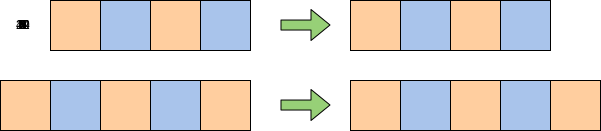Example input (left) and output (right)

Solution and Explanation:

class ProductArray
{
public static int[] findProduct(int arr[])
{
int n = arr.length;
int i, temp = 1;

// Allocation of result array
int result[] = new int[n];

// Product of elements on left side excluding arr[i]
for (i = 0; i < n; i++)
{
result[i] = temp;
temp *= arr[i];
}

// Initializing temp to 1 for product on right side
temp = 1;

// Product of elements on right side excluding arr[i]
for (i = n - 1; i >= 0; i--)
{
result[i] *= temp;
temp *= arr[i];
}

return result;
}
public static String arrayToString(int arr[]){
if (arr.length > 0){
String result = "";
for(int i = 0; i < arr.length; i++) {
result += arr[i] + " ";
}
return result;
}
else {
return "Empty Array!";
}
}

public static void main(String args[]) {

int[] arr = {-1, 2, -3, 4, -5};

System.out.println("Array before product: " + arrayToString(arr));

int[] prodArray = findProduct(arr);

System.out.println("Array after product: " + arrayToString(prodArray));
}
}


Time Complexity: $O(n)$

The algorithm for this solution is to first create a new array with products of all elements to the left of each element, as done on lines 17-20.

Then on lines 27-30, multiply each element in that array to the product of all the elements to the right of the array by traversing it in reverse.

### 7. Remove Even Integers from an Array

Problem Statement:

“In this problem, you have to implement the int [] removeEven(int[] arr) method, which removes all the even elements from the array and returns an updated array. The final array should contain only odd elements with the array reduced in size accordingly.”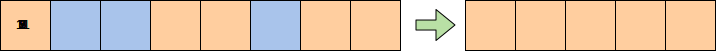Example input (left) and output (right)

Solution and Explanation:

class CheckRemoveEven {

public static int[] removeEven(int[] arr) {
int oddElements = 0;

//Find number of odd elements in arr
for (int i = 0; i < arr.length; i++) {
if (arr[i] % 2 != 0) oddElements++;
}

//Create result array with the size equal to the number of odd elements in arr
int[] result = new int[oddElements];
int result_index = 0;

//Put odd values from arr to the resulted array
for (int i = 0; i < arr.length; i++) {
if (arr[i] % 2 != 0)
result[result_index++] = arr[i];
} //end of for loop

return result;
} //end of removeEven

public static void main(String args[]) {

int size = 10;
int[] arr = new int[size]; //declaration and instantiation

System.out.print("Before removing Even Numbers: ");
for (int i = 0; i < arr.length; i++) {
arr[i] = i; // assigning values
System.out.print(arr[i] +  " ");
}
System.out.println();

int[] newArr =  removeEven(arr); // calling removeEven

System.out.print("After removing Even Numbers: ");
for (int i = 0; i < newArr.length; i++) {
System.out.print(newArr[i] +  " "); // printing array
}
}
}


Time Complexity: $O(n)$

On lines 6 - 9 finds the number of odd elements in the given array by iterating over it and incrementing a count variable if an element is odd.

Next, on lines 11 - 13 we initialize an array with a size oddElements, and store all the odd numbers in it.

Finally, on lines 16 - 19 we fill the array result with all elements from oddElements to return as our solution.

### 8. Remove duplicates from a Linked List

Problem Statement:

“Given a string that contains duplicate occurrences of characters, remove every character that has already appeared previously in the list.”

Solution and Explanation:

class RemoveDuplicates {
// this solution uses extra memory
// to keep all characters present in string.

static void removeDuplicates(char[] str) {
Set<Character> hashset = new LinkedHashSet<Character> ();

int writeIndex = 0;

++writeIndex;
}

}

str[writeIndex] = '\0';
}

// Test Program

static char[] getArray(String t) {
char[] s = new char[t.length() + 1];
int i = 0;
for (; i < t.length(); ++i) {
s[i] = t.charAt(i);
}
s[i] = '\0';
return s;
}

static void print(char[] s) {
int i = 0;
while (s[i] != '\0') {
System.out.print(s[i]);
++i;
}
System.out.println();
}

public static void main(String[] args) {

char[] input = getArray("dabbac");
System.out.print("Before: ");
System.out.println(input);
RemoveDuplicates.removeDuplicates(input);
System.out.print("After: ");
print(input);
}
}


Time Complexity: $O(n)$

In this solution, we’ll keep two pointers, one for the current reading position and one for the current writing position.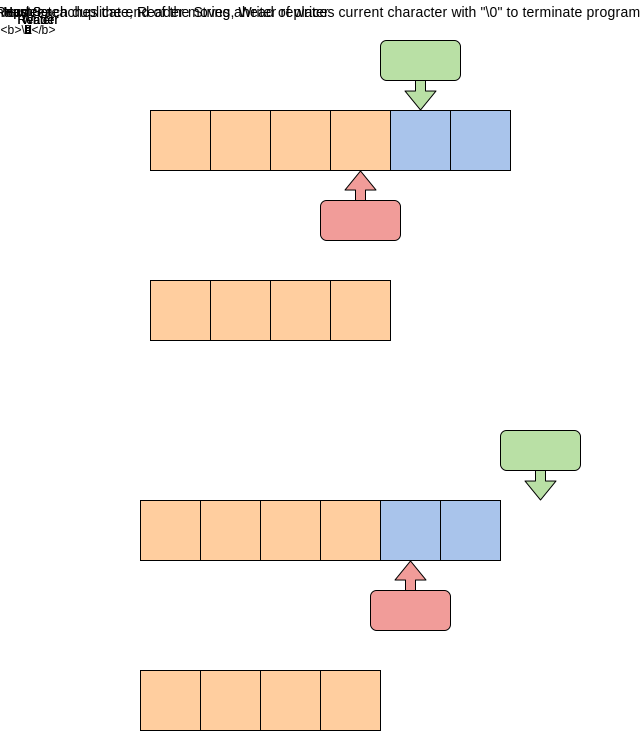Whenever we encounter the first occurrence of a character, we add it to the HashSet. Any character already existing in the HashSet is skipped on any subsequent occurrence.

Below is an overview of the algorithm in pseudocode:

read_pos = 0
write_pos = 0

for each character 'c' in str
write_pos++


### 9. Longest Palindromic Subsequence

Problem Statement:

"Given a sequence, find the length of its Longest Palindromic Subsequence (LPS). In a palindromic subsequence, elements read the same backward and forward.

A subsequence is a sequence that can be derived from another sequence by deleting some or no elements without changing the order of the remaining elements."

Input: "abdbca"
Output: 5
Explanation: LPS is "abdba".


Solution and Explanation:


//brute force
class LPS {

public int findLPSLength(String st) {
return findLPSLengthRecursive(st, 0, st.length()-1);
}

private int findLPSLengthRecursive(String st, int startIndex, int endIndex) {
if(startIndex > endIndex)
return 0;

// every sequence with one element is a palindrome of length 1
if(startIndex == endIndex)
return 1;

// case 1: elements at the beginning and the end are the same
if(st.charAt(startIndex) == st.charAt(endIndex))
return 2 + findLPSLengthRecursive(st, startIndex+1, endIndex-1);

// case 2: skip one element either from the beginning or the end
int c1 =  findLPSLengthRecursive(st, startIndex+1, endIndex);
int c2 =  findLPSLengthRecursive(st, startIndex, endIndex-1);
return Math.max(c1, c2);
}

public static void main(String[] args) {
LPS lps = new LPS();
System.out.println(lps.findLPSLength("abdbca"));
System.out.println(lps.findLPSLength("cddpd"));
System.out.println(lps.findLPSLength("pqr"));
}
}


Time Complexity: $O(2^n)$

A basic brute-force solution could be to try all the subsequences of the given sequence. We can start processing from the beginning and the end of the sequence. So at any step, we have two options:

If the element at the beginning and the end are the same, we increment our count by two and make a recursive call for the remaining sequence.

We will skip the element either from the beginning or the end to make two recursive calls for the remaining subsequence.

If option one applies then it will give us the length of LPS; otherwise, the length of LPS will be the maximum number returned by the two recursive calls from the second option.

Solution 2: Dynamic Programming:

class LPS {

public int findLPSLength(String st) {
Integer[][] dp = new Integer[st.length()][st.length()];
return findLPSLengthRecursive(dp, st, 0, st.length()-1);
}

private int findLPSLengthRecursive(Integer[][] dp, String st, int startIndex, int endIndex) {
if(startIndex > endIndex)
return 0;

// every sequence with one element is a palindrome of length 1
if(startIndex == endIndex)
return 1;

if(dp[startIndex][endIndex] == null) {
// case 1: elements at the beginning and the end are the same
if(st.charAt(startIndex) == st.charAt(endIndex)) {
dp[startIndex][endIndex] = 2 + findLPSLengthRecursive(dp, st, startIndex+1, endIndex-1);
} else {
// case 2: skip one element either from the beginning or the end
int c1 =  findLPSLengthRecursive(dp, st, startIndex+1, endIndex);
int c2 =  findLPSLengthRecursive(dp, st, startIndex, endIndex-1);
dp[startIndex][endIndex] = Math.max(c1, c2);
}
}

return dp[startIndex][endIndex];
}

public static void main(String[] args) {
LPS lps = new LPS();
System.out.println(lps.findLPSLength("abdbca"));
System.out.println(lps.findLPSLength("cddpd"));
System.out.println(lps.findLPSLength("pqr"));
}
}


Time Complexity: $O(n^2)$

We can use an array to store the already solved subproblems.

The two changing values to our recursive function are the two indexes, startIndex and endIndex. Therefore, we can store the results of all the subproblems in a two-dimensional array. (Another alternative could be to use a hash-table whose key would be a string (startIndex + “|” + endIndex))

### 10. Find the Length of a Linked List

Problem Statement:

“Implement the method int length(), which will count the number of nodes in a linked list.”

Solution and Explanation:

main.java
public class SinglyLinkedList<T> {
//Node inner class for SLL
public class Node {
public T data;
public Node nextNode;

}

//constructor
}

public boolean isEmpty() {

if (headNode == null) return true;
return false;
}

//Inserts new data at the start of the linked list
//Creating a new node and assigning it the new data value
Node newNode = new Node();
newNode.data = data;
}

//Inserts new data at the end of the linked list
public void insertAtEnd(T data) {
//if the list is empty then call insertATHead()
if (isEmpty()) {
return;
}
//Creating a new Node with value data
Node newNode = new Node();
newNode.data = data;
newNode.nextNode = null;

//iterate to the last element
while (last.nextNode != null) {
last = last.nextNode;
}
//make newNode the next element of the last node
last.nextNode = newNode;
}

//inserts data after the given prev data node
public void insertAfter(T data, T previous) {

//Creating a new Node with value data
Node newNode = new Node();
newNode.data = data;
//traverse the list until node having data equal to previous is found
while (currentNode != null && currentNode.data != previous) {
currentNode = currentNode.nextNode;
}
//if such a node was found
//then point our newNode to currentNode's nextElement
if (currentNode != null) {
newNode.nextNode = currentNode.nextNode;
currentNode.nextNode = newNode;
}
}

public void printList() {
if (isEmpty()) {
System.out.println("List is Empty!");
return;
}

System.out.print("List : ");

while (temp.nextNode != null) {
System.out.print(temp.data.toString() + " -> ");
temp = temp.nextNode;
}

System.out.println(temp.data.toString() + " -> null");
}

//Searches a value in the given list.
public boolean searchNode(T data) {
//Start from first element

//Traverse the list till you reach end
while (currentNode != null) {
if (currentNode.data.equals(data))
return true; //value found

currentNode = currentNode.nextNode;
}
}

if (isEmpty())
return;
}

public void deleteAtEnd() {
if (isEmpty())
return;
Node currentNode = prevNode.nextNode;
while (currentNode.nextNode != null) {
prevNode = currentNode;
currentNode = currentNode.nextNode;
}
prevNode.nextNode = null;
}

public void deleteByValue(T data) {
//if empty then simply return
if (isEmpty())
return;
Node prevNode = null; //previous node starts from null

if(currentNode.data.equals(data)) {
return;
}

//traverse the list searching for the data to delete
while (currentNode != null) {
//node to delete is found
if (data.equals(currentNode.data)){
prevNode.nextNode = currentNode.nextNode;
return;
}
prevNode = currentNode;
currentNode = currentNode.nextNode;
}
}
public int length() {
//Get the refernce to headNode of the given list
//count is zero initially
int count = 0;
//traverse the list until null is found
while (current!= null){
count++; //increment at each iteration
current = current.nextNode;
}

return count;
}
}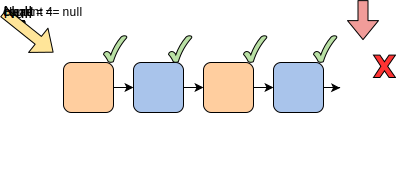Time Complexity: $O(n)$

The trick is to iterate through the list and keep count of how many nodes you’ve visited. This count is held in a variable, count, that is returned when the end of the list is reached.

### 11. Detect Loop in a Linked List

Problem Statement:

"In this problem, you have to implement the public static boolean detectLoop(SinglyLinkedList list) method, which will take a Singly linked list as input and find if there is a loop present in the list. "

A loop in a linked list occurs if any node contains a reference to any previous node, then a loop will be formed.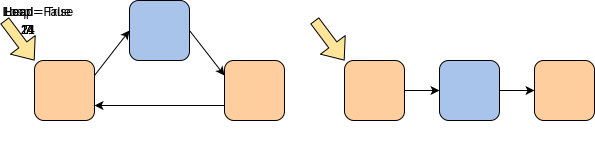Example of a looped vs. non-looped linked lists

You’re provided the following class to use in your solution:

public class SinglyLinkedList<T> {
//Node inner class for SLL
public class Node {
public T data;
public Node nextNode;

}

public int size;

//constructor
size = 0;
}

public boolean isEmpty() {

if (headNode == null) return true;
return false;
}

//Inserts new data at the start of the linked list
//Creating a new node and assigning it the new data value
Node newNode = new Node();
newNode.data = data;
size++;
}

//Inserts new data at the end of the linked list
public void insertAtEnd(T data) {
//if the list is empty then call insertATHead()
if (isEmpty()) {
return;
}
//Creating a new Node with value data
Node newNode = new Node();
newNode.data = data;
newNode.nextNode = null;

//iterate to the last element
while (last.nextNode != null) {
last = last.nextNode;
}
//make newNode the next element of the last node
last.nextNode = newNode;
size++;
}

//inserts data after the given prev data node
public void insertAfter(T data, T previous) {

//Creating a new Node with value data
Node newNode = new Node();
newNode.data = data;
//traverse the list until node having data equal to previous is found
while (currentNode != null && currentNode.data != previous) {
currentNode = currentNode.nextNode;
}
//if such a node was found
//then point our newNode to currentNode's nextElement
if (currentNode != null) {
newNode.nextNode = currentNode.nextNode;
currentNode.nextNode = newNode;
size++;
}
}

public void printList() {
if (isEmpty()) {
System.out.println("List is Empty!");
return;
}

System.out.print("List : ");

while (temp.nextNode != null) {
System.out.print(temp.data.toString() + " -> ");
temp = temp.nextNode;
}

System.out.println(temp.data.toString() + " -> null");
}

//Searches a value in the given list.
public boolean searchNode(T data) {
//Start from first element

//Traverse the list till you reach end
while (currentNode != null) {
if (currentNode.data.equals(data))
return true; //value found

currentNode = currentNode.nextNode;
}
}

//Deletes data from the head of list
//if list is empty then simply return
if (isEmpty())
return;
size--;
}

//Deletes data given from the linked list
public void deleteByValue(T data) {
//if empty then simply return
if (isEmpty())
return;
Node prevNode = null; //previous node starts from null

if(currentNode.data.equals(data)) {
return;
}
//traverse the list searching for the data to delete
while (currentNode != null) {
//node to delete is found
if (data.equals(currentNode.data)){
prevNode.nextNode = currentNode.nextNode;
size--;
return;
}
prevNode = currentNode;
currentNode = currentNode.nextNode;
}
}
}

Solution and Explanation:

main.java
class CheckLoop {

public static <T> boolean detectLoop(SinglyLinkedList<T> list) {

while(slow != null && fast != null && fast.nextNode != null)
{
slow = slow.nextNode;	//the slow pointer will jump 1 step
fast = fast.nextNode.nextNode; //the fast pointer will jump 2 steps
// when the pointers become equal then there must be a loop
if(slow == fast){
return true;
}
}
return false;
}

public static void main( String args[] ) {
System.out.println("Before adding loop: " + detectLoop(list));
System.out.println("After adding loop: " + detectLoop(list));
}
}

Time Complexity: $O(n)$

This is the most optimized method to find out the loop in the LinkedList and uses Floyd’s Cycle Detection Algorithm. We start traversing the LinkedList using two pointers called slow and fast.

Move slow by one (line 9) and fast by two (line 10). If these pointers meet at the same node, then there is a loop. If these pointers do not meet, then LinkedList doesn’t have a loop.

### 12. Implement Two Stacks using one Array

Problem Statement:

“Create class TwoStacks<V> that uses a single array to generate two stacks. Your class will have the following methods:”

void push1(V value)
void push2(V value)
public V pop1()
public V pop2()


 push1(input)

• Input: an integer
• Output: inserts the given value in the first stack i.e. stack1

push2(input)

• Input: an integer
• Output: inserts the given value in the second stack i.e stack2

pop1()

• Output: returns and remove the top value of stack1

pop2()

• Output: returns and remove the top value of stack2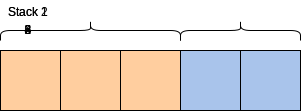An array split into two stacks

You’re supplied the following code to begin your solution:

class TwoStacks<V> {
private int maxSize;
private V[] array;

@SuppressWarnings("unchecked")
public TwoStacks(int max_size) {
this.maxSize = max_size;
array = (V[]) new Object[max_size];//type casting Object[] to V[]
}

//insert at top of first stack
public void push1(V value) {

}

//insert at top of second stack
public void push2(V value) {

}

//remove and return value from top of first stack
public V pop1() {
return null;
}

//remove and return value from top of second stack
public V pop2() {
return null;
}
}


Solution and Explanation:

main.java
TwoStacks.java
class CheckTwoStacks {
public static void main(String args[]) {
TwoStacks<Integer> tS = new TwoStacks<Integer>(5);
tS.push1(11);
tS.push1(3);
tS.push1(7);
tS.push2(1);
tS.push2(9);

System.out.println(tS.pop1());
System.out.println(tS.pop2());
System.out.println(tS.pop2());
System.out.println(tS.pop2());
System.out.println(tS.pop1());
}
}

Time Complexity: $O(1)$

This implementation is space-efficient as it utilizes all of the available space. It doesn’t cause an overflow if there is any space available in the array.

The tops of the two stacks are the two extreme ends of the array. The first stack starts from the first element at index 0, and the second starts from the last element.

The first element in stack2 is pushed at index (maxSize-1). Both stacks grow (or shrink) in the opposite direction.

To check for overflow, all we need do is check for space between the top elements of both stacks, as reflected in the code.

### Reimagine your coding interview prep

Stop grinding through endless practice questions, and start breaking down 100+ real-world problems. Practice as you learn with hands-on coding environments inside your browser. No need to switch to your IDE, download an SDK, or scrub through videos.

Decode the Coding Interview in Java: Real-World Examples

### 13. Level Averages in a Binary Tree

Problem Statement:

“Given a binary tree, populate an array to represent the averages of all of its levels.”

Solution and Explanation:

import java.util.*;

class TreeNode {
int val;
TreeNode left;
TreeNode right;

TreeNode(int x) {
val = x;
}
};

class LevelAverage {
public static List<Double> findLevelAverages(TreeNode root) {
List<Double> result = new ArrayList<>();
if (root == null)
return result;

queue.offer(root);
while (!queue.isEmpty()) {
int levelSize = queue.size();
double levelSum = 0;
for (int i = 0; i < levelSize; i++) {
TreeNode currentNode = queue.poll();
// add the node's value to the running sum
levelSum += currentNode.val;
// insert the children of current node to the queue
if (currentNode.left != null)
queue.offer(currentNode.left);
if (currentNode.right != null)
queue.offer(currentNode.right);
}
// append the current level's average to the result array
}

return result;
}

public static void main(String[] args) {
TreeNode root = new TreeNode(12);
root.left = new TreeNode(7);
root.right = new TreeNode(1);
root.left.left = new TreeNode(9);
root.left.right = new TreeNode(2);
root.right.left = new TreeNode(10);
root.right.right = new TreeNode(5);
List<Double> result = LevelAverage.findLevelAverages(root);
System.out.print("Level averages are: " + result);
}
}


Time Complexity: $O(n)$

This problem follows the Binary Tree Level Order Traversal pattern. We can follow the same BFS approach.

The only difference will be that instead of keeping track of all nodes of a level, we will only track the running sum of the values of all nodes in each level.

In the end, we will append the average of the current level to the result array.

### 14. Binary Tree Path Sum

Problem Statement:

"Given a binary tree and a number S, find if the tree has a path from root-to-leaf such that the sum of all the node values of that path equals S. "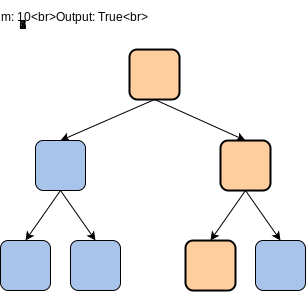Highlighted Path that sums to 10

Solution and Explanation:

class TreeNode {
int val;
TreeNode left;
TreeNode right;

TreeNode(int x) {
val = x;
}
};

class TreePathSum {
public static boolean hasPath(TreeNode root, int sum) {
if (root == null)
return false;

// if the current node is a leaf and its value is equal to the sum, we've found a path
if (root.val == sum && root.left == null && root.right == null)
return true;

// recursively call to traverse the left and right sub-tree
// return true if any of the two recursive call return true
return hasPath(root.left, sum - root.val) || hasPath(root.right, sum - root.val);
}

public static void main(String[] args) {
TreeNode root = new TreeNode(12);
root.left = new TreeNode(7);
root.right = new TreeNode(1);
root.left.left = new TreeNode(9);
root.right.left = new TreeNode(10);
root.right.right = new TreeNode(5);
System.out.println("Tree has path: " + TreePathSum.hasPath(root, 23));
System.out.println("Tree has path: " + TreePathSum.hasPath(root, 16));
}
}


Time Complexity: $O(n)$

As we are trying to search for a root-to-leaf path, we can use the Depth First Search (DFS) technique to solve this problem.

To recursively traverse a binary tree in a DFS fashion, we can start from the root and at every step, make two recursive calls one for the left and one for the right child.

Here are the steps for our Binary Tree Path Sum problem:

1. Start DFS with the root of the tree.
2. If the current node is not a leaf node, do two things:
• Subtract the value of the current node from the given number to get a new sum => S = S - node.value
• Make two recursive calls for both the children of the current node with the new number calculated in the previous step.
3. At every step, see if the current node being visited is a leaf node and if its value is equal to the given number S. If both these conditions are true, we have found the required root-to-leaf path, therefore return true.
4. If the current node is a leaf but its value is not equal to the given number S, return false.

### 15. Find All of the Words in a Trie

Problem Statement:

“Implement the findWords() function that returns all of the words stored in a Trie in alphabetically sorted order. There are 9 words total in the given keys array, so we just need to iterate the Trie and return all of the words present in it.”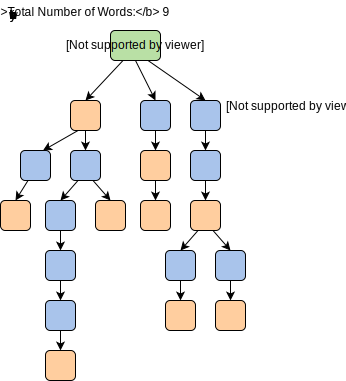Solution and Explanation:

main.java
TrieNode.java
Trie.java
class TrieWords {
//Recursive Function to generate all words
public static void getWords(TrieNode root, ArrayList < String > result, int level, char[] str) {
//Leaf denotes end of a word
if (root.isEndWord) {
//current word is stored till the 'level' in the character array
String temp = "";
for (int x = 0; x < level; x++) {
temp += Character.toString(str[x]);
}
}
for (int i = 0; i < 26; i++) {
if (root.children[i] != null) {
//Non-null child, so add that index to the character array
str[level] = (char)(i + 'a');
getWords(root.children[i], result, level + 1, str);
}
}
}
public static ArrayList < String > findWords(TrieNode root) {
ArrayList < String > result = new ArrayList < String > ();
char[] chararr = new char;
getWords(root, result, 0, chararr);
return result;
}

public static void main(String args[]) {
// Input keys (use only 'a' through 'z' and lower case)
String keys[] = {"the", "a", "there", "answer", "any",
"by", "bye", "their","abc"};
String output[] = {"Not present in trie", "Present in trie"};
Trie t = new Trie();

System.out.println("Keys: "+ Arrays.toString(keys));

// Construct trie

for (int i = 0; i < keys.length ; i++)
t.insert(keys[i]);

ArrayList < String > list = findWords(t.getRoot());
for(int i = 0; i < list.size(); i++) {
System.out.println(list.get(i));
}

}
}

Time Complexity: $O(n)$

The findWords(root) function contains a result ArrayList which will contain all the words in the trie. word is a character array in which node characters are added one by one to keep track of all the alphabets in the same recursive call.

getWords() is our recursive function which begins from the root and traverses every node. Whenever a node is the end of a word, temp (containing the character array) is converted into a string and inserted into result.

Since word cannot be reset before recording every new word, we simply update the values at each index using level.

## Recursion

### 16. Find the Greatest Common Divisor

Problem Statement:

“Write a recursive method that computes the Greatest Common Divisor of two integers.”

The GCD of two integers is the largest integer that can fully divide both numbers without a remainder.

• The method should take two integers as input.
• Their GCD is to be computed, as input.
• The method should return the GCD of the two integers as output.
• The method should be recursive.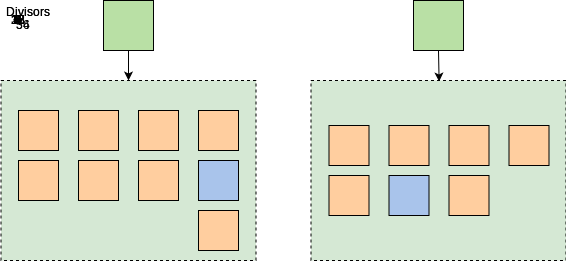Example: GCD = 18

Solution and Explanation:

class Solution {

public static int gcd(int num1, int num2) {

// Base case
if (num1 == num2) {
return num1;
}
// Recursive case
if (num1 > num2) {
return gcd(num1-num2, num2);
}
else {
return gcd(num1, num2-num1);
}
}

public static void main( String args[] ) {
int x = 56;
int y = 42;
int result = gcd(x, y);
System.out.println("The GCD of " + x + " and " + y + " is:");
System.out.println(result);
}
}


Recursive Method

The return type of this method is an integer because GCD of two numbers is always an integer.

The method takes two integer input arguments num1 and num2.

Base Case

The base case of the method is defined in the if block, from line 6 - 8.

When num2 is equal to num1, the method returns num1.

Recursive Case

If the base case does not compute to be true, the method checks the second if condition on line 10, to be true i.e if num1 > num2.

If it computes to true, a recursive call is made with parameters num1-num2 and num2 respectively.

If the base case and the if condition does not compute to true, the method automatically goes to the else statement on line 14, where num2 > num1 and a recursive call is made with parameters num1 and num2-num1 respectively.

In the case above the recursive calls will be as follows:

First recursive call will be for num2 > num1 and will be gcd(56-42,42).

Second recursive call will be for num1 > num2 and will be gcd(14,42-14).

Third recursive call will be for num1 > num2 and will be gcd(14,28-14).

After the third recursive call, the num1==num2 condition computes to true.

So, the base case is reached and the method returns num1, which is equal to $14$.

### 17. Check for Prime Number

Problem Statement:

“Write a recursive method, named isPrime() that checks if a number is prime or not.”

• The method should take two integers as input.
• The method should return Boolean, meaning it will return true if the number is prime or return false if the number is not prime.
• The method should be recursive.

Solution and Explanation:

class ChallengeClass {

public static boolean isPrime(int num, int i) {

// First base case
if (num < 2) {
return false;
}
// Second base case
else if (i == 1) {
return true;
}
// Third base case
else if (num%i == 0) {
return false;
}
// Recursive case
else {
return isPrime(num, i-1);
}
}

public static void main( String args[] ) {
int input = 13;
boolean result = isPrime(input, input/2);

// Print if the number is prime
if (result == true) {
System.out.println(input + " is a prime number");
}
// Prints if the number is NOT a prime number
else {
System.out.println(input + " is NOT a prime number");
}
}
}

Recursive Method

The return type of this method is Boolean since we want to check if the number is prime or not and it takes the two integers, num and i, as input parameters.

num is the number to be tested and i is the iterator and has the initial value of num/2 when the method is called in the main code.

i has the initial value of num/2 because all the factors of a number are less than equal to the half of that number. We iterate through all the numbers less than or equal to i to check if num has a factor. We use the % operator to check for the factors of num.

Base Case

We have defined the base cases for the method in the if conditions.

In the first if condition, line 6-8, when num is less than or equal to $2$, the method returns num since $0$ and $1$ are not prime numbers.

In the second if condition, lines 10-12, when i is equal to $1$, the method returns true. We will need to understand the recursive part of the method to understand this base case.

In the third if condition, lines 14-16, if the number has a divisor other than $1$, the method returns false. To check for this condition, we use the % operator to compute modulo of num with the iterator i.

Recursive Case

When the if condition for any of the base cases does not compute to be true, the method enters the else block, lines 18-20, where it makes a recursive call.

In the recursive call, the isPrime() method is called with the first parameter, num, and the second parameter, i-1.

In this case, the recursive calls will be as follows:

• The first recursive call will be isPrime(13,5).

• The second recursive call will be isPrime(13,4).

• The third recursive call will be isPrime(13,3).

• The fourth recursive call will be isPrime(13,2).

• The fifth recursive call will be isPrime(13,1) which is when the second base case condition will be reached since i==1.

The method will return true since num is divisible by $1$ only.

### 18. Recursive Insert into Binary Search Tree

Problem Statement:

“Create a recursive method that inserts an element at it’s appropriate position in a binary search tree.”

Solution and Explanation:

class binarySearchTree {

//Variables
private Node root;
//Getter for Root
public Node getRoot() {
return root;
}
//Setter for root
public void setRoot(Node root) {
this.root = root;
}

//Recursive function to insert a value in BST
public Node recursive_insert(Node currentNode, int value) {

//Base Case
if (currentNode == null) {
return new Node(value);
}

if (value < currentNode.getData()) {
//Iterate left sub-tree
currentNode.setLeftChild(recursive_insert(currentNode.getLeftChild(), value));
} else if (value > currentNode.getData()) {
//Iterate right sub-tree
currentNode.setRightChild(recursive_insert(currentNode.getRightChild(), value));
} else {
return currentNode;
}

return currentNode;
}

//Function to call recursive insert
public boolean insert(int value) {

root = recursive_insert(this.root, value);
return true;
}

//Function to check if Tree is empty or not
public boolean isEmpty() {
return root == null; //if root is null then it means Tree is empty
}

//Just for Testing purpose
public void printTree(Node current) {

if (current == null) return;

System.out.print(current.getData() + ",");
printTree(current.getLeftChild());
printTree(current.getRightChild());

}
public static void main(String args[]) {

binarySearchTree bsT = new binarySearchTree();
bsT.insert(6);
bsT.insert(4);
bsT.insert(8);
bsT.insert(5);
bsT.insert(2);
bsT.insert(8);
bsT.insert(12);
bsT.insert(10);
bsT.insert(14);
bsT.printTree(bsT.getRoot());

}
}


Recursive Method

The return type of the insert() function is boolean and takes one integer type of input parameter value which depicts the value of the node.

This method calls the actual recursive method recursive_insert that takes two input parameters. The first is currentNode of type Node. The second parameter value is the integer to be inserted in the BST. We will discuss the recursive_insert method below.

Base Case

We have defined a base case for the method in first if condition on lines 19 - 21.

If the value of the currentNode is null, meaning there is an available space for the child node to be inserted, a new Node() is created with the value.

Recursive Case

If the base case condition is not met and the value to be inserted is less than the value of currentNode.getData(), the function enters the second if condition, line 25, where it makes a recursive call.

In this process, the recursive_insert method is recursive. The first parameter is currentNode.getLeftChild() and the second parameter is value.

If the method has not reached the base and the inserted value is greater than the value of currentNode.getData(), the function enters the other else if condition, in line 26-28, where it makes a recursive call.

In this else if condition, the recursive_insert method is called. The first parameter is currentNode.getRightChild() and the second parameter is value.

If all the above conditions are not met, we reach the else condition from lines 29 to 31 which returns the currentNode since the defined value already exists.

Line 34 returns the currentNode after being inserted into its position.

Subsequent recursive calls will continually be made until the first parameter equals null, which ensures the availability of a position for the new node. The new node is then added to its corresponding position.

## Dynamic Programming

### 19. Remove duplicates from a Linked List

Problem Statement:

“Given a number array to represent different coin denominations and a total amount T, find all the different ways to make change for T with the given coin denominations. We can assume an infinite supply of coins, therefore, each coin can be chosen multiple times.”

Solution and Explanation:

A basic brute-force solution could be to try all combinations of the given coins to select the ones that give a total sum of T. Here is what that would look like in pseudocode:

for each coin 'c'
create a new set which includes one quantity of coin 'c' if it does not exceed 'T', and
recursively call to process all coins
create a new set without coin 'c', and recursively call to process the remaining coins
return the count of sets who have a sum equal to 'T'


We can instead use Top-Down Dynamic Programming with Memoization to optimize our solution.

We can use memoization to overcome the overlapping sub-problems. We will be using a two-dimensional array to store the results of solved sub-problems. We need to store results for every coin combination and for every possible sum.

class CoinChange {

public int countChange(int[] denominations, int total)
{
Integer[][] dp = new Integer[denominations.length][total + 1];
return this.countChangeRecursive(dp, denominations, total, 0);
}

private int countChangeRecursive(Integer[][] dp, int[] denominations, int total, int currentIndex)
{
// base checks
if (total == 0)
return 1;

if(denominations.length == 0 || currentIndex >= denominations.length)
return 0;

// if we have already processed a similar sub-problem, return the result from memory
if(dp[currentIndex][total] != null)
return dp[currentIndex][total];

// recursive call after selecting the coin at the currentIndex
// if the number at currentIndex exceeds the total, we shouldn't process this
int sum1 = 0;
if( denominations[currentIndex] <= total )
sum1 = countChangeRecursive(dp, denominations, total - denominations[currentIndex], currentIndex);

// recursive call after excluding the number at the currentIndex
int sum2 = countChangeRecursive(dp, denominations, total, currentIndex + 1);

dp[currentIndex][total] = sum1 + sum2;
return dp[currentIndex][total];
}

public static void main(String[] args) {
CoinChange cc = new CoinChange();
int[] denominations = {1, 2, 3};
System.out.println(cc.countChange(denominations, 5));
}
}


Time Complexity: $O(C \times T)$

### 20. Minimum jumps to reach the end

Problem Statement:

“Given an array of positive numbers, where each element represents the max number of jumps that can be made forward from that element, write a program to find the minimum number of jumps needed to reach the end of the array (starting from the first element). If an element is 0, then we cannot move through that element.”

// Example input and output

Input = {2,1,1,1,4}
Output = 3
Explanation: Starting from index '0', we can reach the last index through: 0->2->3->4Visual of jumps through input array

Solution and Explanation:

We will start with the 0th index and try all options. So, if the value at the current index is p, we will try every jump in the range (1 to p) from that index. After taking a jump, we recursively try all options from that index.

However, we can optimize this solution with a bottom up approach.

Let’s try to populate our dp[] array from the above solution, working in the bottom-up fashion. As we saw in the above code, we were trying to find the minimum jumps needed to reach every index (if it is within the range) from the current index. We can use this fact to populate our array.

As we know, every index within the range of the current index can be reached in one jump. Therefore, we can say that we can reach every index (within the range of current index) in:

    'jumps to reach current index' + 1


So, while going through all the indexes, we will take the minimum value between the current jump-count and the jumps needed to reach the current index + 1.

class ArrayJump {

public int countMinJumps(int[] jumps) {
int[] dp = new int[jumps.length];

//initialize with infinity, except the first index which should be zero as we start from there
for(int i=1; i<jumps.length; i++)
dp[i] = Integer.MAX_VALUE;

for(int start=0; start < jumps.length-1; start++) {
for(int end=start+1; end <= start+jumps[start] && end < jumps.length; end++)
dp[end] = Math.min(dp[end], dp[start]+1);
}

return dp[jumps.length-1];
}

public static void main(String[] args) {
ArrayJump aj = new ArrayJump();
int[] jumps = {2, 1, 1, 1, 4};
System.out.println(aj.countMinJumps(jumps));
jumps = new int[]{1, 1, 3, 6, 9, 3, 0, 1, 3};
System.out.println(aj.countMinJumps(jumps));
}
}
`

Time Complexity: $O(n^2)$

## 20 More Mixed Questions

1. Egg Drop Problem
2. Maximum Ribbon Cut Problem
3. Staircase Problem
4. 0/1 Knapsack Problem
5. Interleaving Strings
6. Longest Repeating Subsequence
7. House Thief Problem
8. Depth First Search in Graph
9. First Non-repeating Integer in an Array
10. Cyclic Sort
1. Bitonic Array Maximum
2. Triplet Sum to Zero
3. Search in a Sorted Infinite Array
4. Reverse every K Element Sub-list in a Linked List
6. Intersection Point of Two Linked Lists
7. Boggle Problem
8. Check for Balanced Parentheses using Stack
9. Print Tree Perimeter
10. Sliding Window Median

## Wrapping up and next steps

Congratulations on finishing those 40 questions! The best way to prepare for coding interviews is the practice you’re doing right now. Soon, you’ll know all the question types you could encounter at your next interview.

To help you prepare for interviews, Educative has created the unique course Decode the Coding Interview in Java: Real-World Examples. Available in multiple languages, this course takes you through 100+ real-world questions like these. After each project, you’ll better understand what techniques you just applied.

This is coding interview prep reimagined, with your needs in mind.

Happy learning!